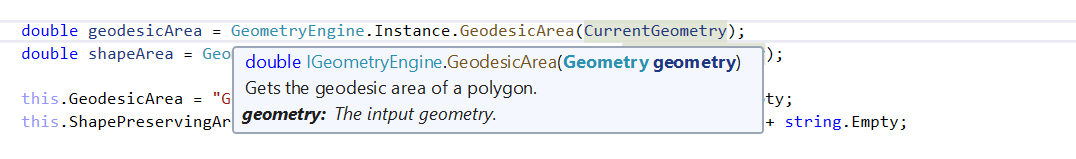# How do I manipulate with specific linear unit in GeometryEngine.Instance function

270
2
Jump to solution
01-27-2020 07:19 PMbyEsri Regular Contributor

Hi Guys,

How do I manipulate with specific linear unit in GeometryEngine.Instance at specific function.

For example. GeometryEngine.Instane.GeodesicArea([input Geometry), the output seem/support to be derived from SpatialReference unit of geometry. For example, If I want to get output with meter, how should I manipulate.

Is that require to reproject with WGS 84 whenever I want to calculate in meter?

Same thing for

GeometryEngine.Instance.GeodesicBuffer, GeometryEngine.Instance.Buffer, => will the distance value derived from spatialreference of the input geometry?

For example. If I want to buffer in kilometer how should I do?

I found some function with the option linear unit in arcgis runtime sdk .net Geodesic Buffer | ArcGIS for Developers

I think, that could be great if these .net libraries are using the common data transfer object dll.

Tags (1)
1 Solution

Accepted SolutionsNew Contributor III

Hi,

The documentation should say what the input and output units are.

GeodesicArea always returns square meters. You don't need to project your data.

ArGIS Pro 2.4 API Reference Guide - GeodesicArea

GeodesicBuffer assumes the distance passed in is in meters.

ArcGIS Pro 2.4 API Reference Guide - GeodesicBuffer

If you need different units, use the LinearUnit class to convert them. For example, if you want to buffer in kilometers instead of meters, convert the distance from kilometers to meters and then pass that to the GeodesicBuffer method.

``LinearUnit kilometers = LinearUnit.Kilometers;double meters = kilometers.ConvertTo(100, LinearUnit.Kilometers);Geometry buffer = GeometryEngine.Instance.GeodesicBuffer(polygon, meters);‍‍‍``

That being said, we can add methods with a LinearUnit parameter in a future release. Thanks for the suggestion.

Annette

2 RepliesNew Contributor III

Hi,

The documentation should say what the input and output units are.

GeodesicArea always returns square meters. You don't need to project your data.

ArGIS Pro 2.4 API Reference Guide - GeodesicArea

GeodesicBuffer assumes the distance passed in is in meters.

ArcGIS Pro 2.4 API Reference Guide - GeodesicBuffer

If you need different units, use the LinearUnit class to convert them. For example, if you want to buffer in kilometers instead of meters, convert the distance from kilometers to meters and then pass that to the GeodesicBuffer method.

``LinearUnit kilometers = LinearUnit.Kilometers;double meters = kilometers.ConvertTo(100, LinearUnit.Kilometers);Geometry buffer = GeometryEngine.Instance.GeodesicBuffer(polygon, meters);‍‍‍``

That being said, we can add methods with a LinearUnit parameter in a future release. Thanks for the suggestion.

AnnettebyEsri Regular Contributor

Thank Annette Locke‌,

So it  look like there are two parts, area, distance, distance3d shall be based on geometry spatial reference.

Geodesic, shape preserving .. shall be meter based.

Would like to suggest one more item, can you please add return type info into the summary comment of the methods as well?

Visual studio 2017/2019 seem does not fix it well, without resharper, I cannot see the return type.For example like below.

``///         <summary>Gets the geodesic area of a polygon. The area returned is in square meters.</summary><param name="geometry">The intput geometry.</param><exception cref="T:System.ArgumentNullException">Geometry is null or empty.</exception><exception cref="T:System.NotImplementedException">The method is not implemented for GeometryBag or Multipatch.</exception><exception cref="T:ArcGIS.Core.Geometry.GeometryObjectException">Geometry is of the wrong type (i.e. a line).</exception><exception cref="T:System.NotImplementedException">        ///   Spatial reference of <paramref name="geometry" />is an image coordinate system.        /// </exception><returns>        ///   The calculated geodesic area. The area returned is in square meters.        /// </returns>public double GeodesicArea(Geometry geometry){}‍‍‍‍‍‍‍‍‍``

Best Regards,